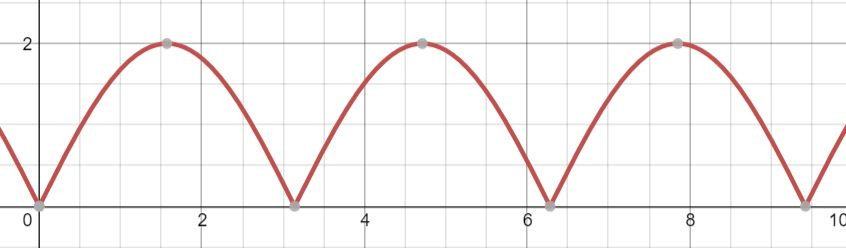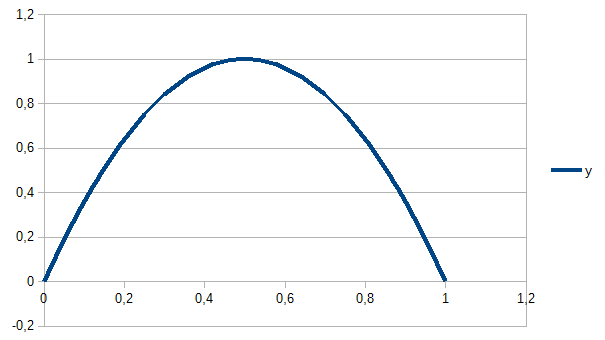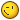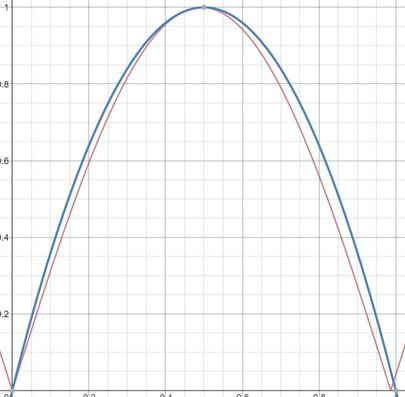# Bouncing a sprite

## Recommended Posts

I'm trying to bounce a sprite so that its y coordinate follows a path like this;The animation should accelerate as it is closer to the origin, and decelerate at the apex.  This function is in fact;

`abs(sin(x) * 2)`

I'm trying to do this in phaser using Easing.Quadratic.In with yoyo=true, however the animation slows at both the origin and the apex.

Any ideas on how I can achieve this?

I guess I could set the position inside an update using the function above instead of tweening, is there any performance difference between doing that or using Phaser.Tween?

##### Share on other sites

Hi, what you need is not abs(sin(x)) but parabolic.

Phaser tween easing functions take value from zero to 1 and return result, which is usually 0 in the beginning and 1 in the end. In your case you want to return 0 in the beginning and end too. So, best way for you is to write custom easing function, that will produce parabolic curve as on image below. This function (y) is 0 in time 0 (x) and 0 again in end of tween time (which is 1). In time 0,5 it is 1.

Parabolic curve has equation: y = ax^2 + bx + c. You know three points [x, y], so you get three equations:

• 0 = a0^2 + b0 + c = c
• 0 = a1^2 + b + c = a + b + c
• 1 = a0.5^2 + 0.5b + c

from first c = 0 and from other two you can calculate a and b: a = -4; b = 4

Your eqaution for easing function is: y = -4x^2 + 4x + 0Now, some code: you will need two tweens - first one for y parabolic movement (like jumping on place) and second for linear movement along x:

```            this.game.add.tween(mySprite).to({ y: -200 }, 1000, function (k) {
return -4 * k * k + 4 * k + 0;
}, true, 0, 2);

this.game.add.tween(mySprite).to({ x: 250 }, 3000, Phaser.Easing.Linear.None, true, 0);```

First tween takes 1 second (1000 millis) and is repeated 3 times (2 as last param).

Second tween takes 3 seconds and 1 repeat.

It all results into three tweened jumps and then it stops. Note, I have 0,0 in center of screen. If you have 0,0 in top left corner (default) then you will have to change y in first tween to see something on screen...

Btw: I wrote article on custom easing functions on my blog: http://sbcgamesdev.blogspot.cz/2015/04/phaser-tutorial-custom-easing-functions.html There is much more complex bouncing function (with elasticity, gravity, ...).

##### Share on other sites

23 minutes ago, Tom Atom said:

what you need is not abs(sin(x)) but parabolic.

Turns out that the following is true for 0 <= x <= 1;

`Math.abs(Math.sin(10 * k / Math.PI)) === -4 * k * k + 4 * k`

So I can use sin(), however the gotcha occurred when you pointed out that the tween should return a value between 0 and 1.  This allowed me to determine that

1. Using yoyo=true was incorrect and;
2. I needed to reduce my equation to output values 0 => y >= 1

Thanks for your help Tom, I'm having a read through your blog now##### Share on other sites

23 minutes ago, staff0rd said:

Turns out that the following is true for 0 <= x <= 1;

```
Math.abs(Math.sin(10 * k / Math.PI)) === -4 * k * k + 4 * k```

So I can use sin(), however the gotcha...

What? Sine is not parabolic, you can approximate a sine curve within some range with a polynomial but it is never exact

##### Share on other sites

30 minutes ago, chg said:

What? Sine is not parabolic, you can approximate a sine curve within some range with a polynomial but it is never exact

You are right; blue is the parabola, red sine;My bad, my highschool maths was so long ago.  Hard to notice the difference in a tween however.  I wonder if the performance suffers?